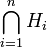# Intersection of finitely many verbal subgroups

Jump to: navigation, search
This article defines a subgroup property: a property that can be evaluated to true/false given a group and a subgroup thereof, invariant under subgroup equivalence. View a complete list of subgroup properties[SHOW MORE]

## Definition

Suppose$G$ is a group and$H$ is a subgroup of$G$. We say that$H$ is an intersection of finitely many verbal subgroups in$G$ if there exists a positive integer$n$ and verbal subgroups$H_1,H_2,\dots,H_n$ of$G$ such that$H$ equals the intersection of subgroups$\bigcap_{i=1}^n H_i$.

## Relation with other properties

### Stronger properties

Property Meaning Proof of implication Proof of strictness (reverse implication failure) Intermediate notions
verbal subgroup generated by a set of words |FULL LIST, MORE INFO

### Weaker properties

Property Meaning Proof of implication Proof of strictness (reverse implication failure) Intermediate notions
existentially bound-word subgroup |FULL LIST, MORE INFO
fully invariant subgroup invariant under all endomorphisms |FULL LIST, MORE INFO
characteristic subgroup invariant under all automorphisms Fully invariant subgroup|FULL LIST, MORE INFO
normal subgroup invariant under all inner automorphisms Fully invariant subgroup|FULL LIST, MORE INFO